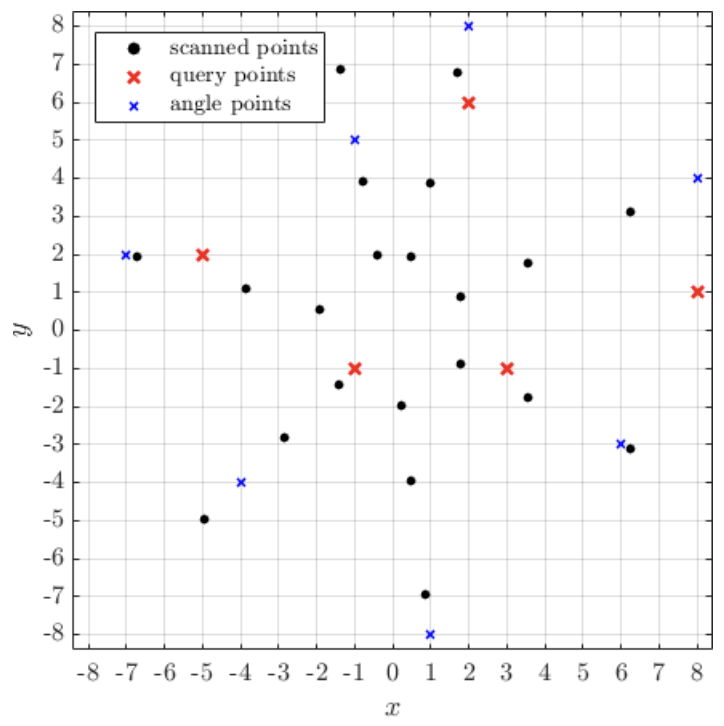시간 제한메모리 제한제출정답맞힌 사람정답 비율
2 초 256 MB59000.000%

## 문제

We are using a special radar to scan an area. The radar accepts a list of distances, e.g. $2$, $4$, $1$, and a list of angles, e.g. $100^◦$, $270^◦$, $180^◦$, $10^◦$, $300^◦$, and scans the points across all the given distances and angles. How close to some other points of interest will we be able to scan?

## 입력

The first line of the input gives three space-separated integers: $R$, $F$, $N$, representing the number of radii, the number of angles, and the number of points of interest, respectively. Then $R$ lines follow, $i$-th of which contains an integer $r_i$, representing the distance from the radar that will be scanned. Then, $F$ lines follow, each containing two space-separated integers $(f_x)_i$, $(f_y)_i$, that represent Cartesian coordinates of a point, defining the $i$-th angle. Then, $N$ lines follow, each containing two space-separated integers $x_i$, $y_i$, that represent the Cartesian coordinates of the $i$-th point.

The angle, defined by the point $(f_x)_i$, $(f_y)_i$ is the angle from the $x$-axis to the ray from the origin through $(f_x)_i$, $(f_y)_i$.

## 출력

Output $N$ lines, $i$-th of which should contain the distance from the point $(x_i , y_i)$ to the closest scanned point. The result will be considered correct if it is within the $10^{-6}$ of absolute or relative precision.

## 제한

• $1 ≤ R, F, N ≤ 10^5$
• $|x_i|, |y_i|, |(f_x)_i|, |(f_y)_i|, r_i < 10^6$
• $(f_x)^2_i + (f_y)^2_i , r_i > 0$
• All $r_i$ are pairwise distinct.
• Rays, defined by $(f_x)_i$,$(f_y)_i$, are pairwise distinct.

## 예제 입력 1

3 7 5
2
4
7
8 4
2 8
-1 5
-7 2
-4 -4
1 -8
6 -3
3 -1
8 1
2 6
-5 2
-1 -1


## 예제 출력 1

0.977772290466
2.750120773895
0.846777708005
1.464071052924
0.585786437627


## 힌트

Illustration of sample case: